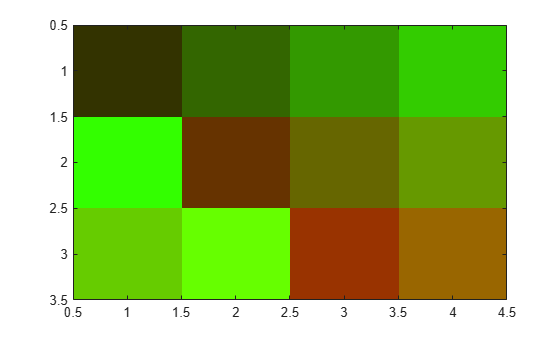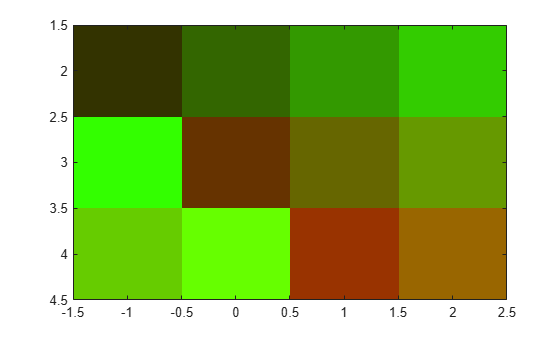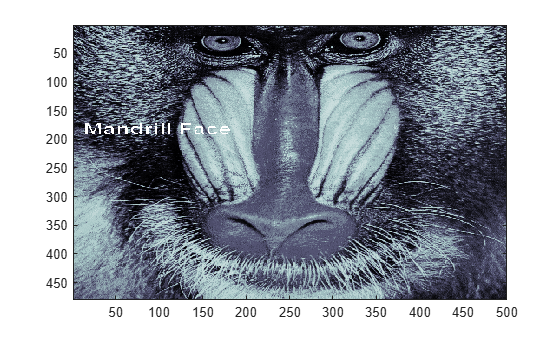## The Image Object and Its Properties

### Note

The `image` and `imagesc` commands create image objects. Image objects are children of axes objects, as are line, patch, surface, and text objects. Like all graphics objects, the image object has a number of properties you can set to fine-tune its appearance on the screen. The most important properties of the image object with respect to appearance are `CData`, `CDataMapping`, `XData`, and `YData`. These properties are discussed in this and the following sections. For detailed information about these and all the properties of the image object, see `image`.

The `CData` property of an image object contains the data array. In the following commands, `h` is the handle of the image object created by `image`, and the matrices `X` and `Y` are the same:

```h = image(X); colormap(map) Y = get(h,'CData'); ```

The dimensionality of the `CData` array controls whether the image displays using colormap colors or as an RGB image. If the `CData` array is two-dimensional, the image is either an indexed image or an intensity image; in either case, the image is displayed using colormap colors. If, on the other hand, the `CData` array is m-by-n-by-3, it displays as a truecolor image, ignoring the colormap colors.

### Image CDataMapping

The `CDataMapping` property controls whether an image is `indexed` or `intensity`. To display an indexed image set the `CDataMapping` property to `'direct'`, so that the values of the `CData` array are used directly as indices into the figure's colormap. When the `image` command is used with a single input argument, it sets the value of `CDataMapping` to `'direct'`:

```h = image(X); colormap(map) get(h,'CDataMapping') ans = direct ```

Intensity images are displayed by setting the `CDataMapping` property to `'scaled'`. In this case, the `CData` values are linearly scaled to form colormap indices. The axes `CLim` property controls the scale factors. The `imagesc` function creates an image object whose `CDataMapping` property is set to `'scaled'`, and it adjusts the `CLim` property of the parent axes. For example:

```h = imagesc(I,[0 1]); colormap(map) get(h,'CDataMapping') ans = scaled get(gca,'CLim') ans = [0 1] ```

### XData and YData

The `XData` and `YData` properties control the coordinate system of the image. For an m-by-n image, the default `XData` is `[1 n]` and the default `YData` is `[1 m]`. These settings imply the following:

• The left column of the image has an x-coordinate of 1.

• The right column of the image has an x-coordinate of n.

• The top row of the image has a y-coordinate of 1.

• The bottom row of the image has a y-coordinate of m.

#### Coordinate System for Images

Use Default Coordinate System

Display an image using the default coordinate system. Use colors from the `colorcube` map.

```C = [1 2 3 4; 5 6 7 8; 9 10 11 12]; im = image(C); colormap(colorcube)```Specify Coordinate System

Display an image and specify the coordinate system. Use colors from the `colorcube` map.

```C = [1 2 3 4; 5 6 7 8; 9 10 11 12]; x = [-1 2]; y = [2 4]; figure image(x,y,C) colormap(colorcube)```### Add Text to Image Data

This example shows how to use array indexing to rasterize text into an existing image.

Draw the text in an axes using the `text` function. Then, capture the text from the screen using `getframe` and close the figure.

```fig = figure; t = text(.05,.1,'Mandrill Face','FontSize',20,'FontWeight','bold'); F = getframe(gca,[10 10 200 200]); close(fig)```

Select any plane of the resulting RGB image returned by `getframe`. Find the pixels that are black (black is 0) and convert their subscripts to indexes using `sub2ind`. Use these subscripts to "paint" the text into the image contained in the `mandrill` MAT-file. Use the size of that image, plus the row and column locations of the text to determine the locations in the new image. Index into new image, replacing pixels.

```c = F.cdata(:,:,1); [i,j] = find(c==0); load mandrill ind = sub2ind(size(X),i,j); X(ind) = uint8(255);```

Display the new image using the bone colormap.

```imagesc(X) colormap bone```### Additional Techniques for Fast Image Updating

To increase the rate at which the `CData` property of an image object updates, optimize `CData` and set some related figure and axes properties:

• Use the smallest data type possible. Using a `uint8` data type for your image will be faster than using a `double` data type.

Part of the process of setting the image's `CData` property includes copying the matrix for the image's use. The overall size of the matrix is dependent on the size of its individual elements. Using smaller individual elements (i.e., a smaller data type) decreases matrix size, and reduces the amount of time needed to copy the matrix.

• Use the smallest acceptable matrix.

If the speed at which the image is displayed is your highest priority, you may need to compromise on the size and quality of the image. Again, decreasing the size reduces the time needed to copy the matrix.

• Set the limit mode properties (`XLimMode` and `YLimMode`) of your axes to `manual`.

If they are set to `auto`, then every time an object (such as an image, line, patch, etc.) changes some aspect of its data, the axes must recalculate its related properties. For example, if you specify

```image(firstimage); set(gca, 'xlimmode','manual',... 'ylimmode','manual',... 'zlimmode','manual',... 'climmode','manual',... 'alimmode','manual'); ```

the axes do not recalculate any of the limit values before redrawing the image.

• Consider using a `movie` object if the main point of your task is to simply display a series of images onscreen.

The MATLAB® `movie` object utilizes underlying system graphics resources directly, instead of executing MATLAB object code. This is faster than repeatedly setting an image's `CData` property, as described earlier.